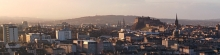# Machine Learning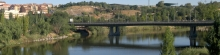## Malaria Parasite Detection Using an SVM

Recently I read the excellent tutorial Deep Learning and Medical Image Analysis with Keras by Dr. Adrian Rosebrock’s. Adrian’s tutorial shows how to use a pr...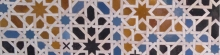## CIFAR-10 Classifier Using CNN in PyTorch

In this notebook we will use PyTorch to construct a convolutional neural network. We will then train the CNN on the CIFAR-10 data set to be able to classify ...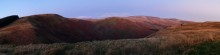## Tensors and Gradients in PyTorch

In this notebook we will learn what tensors are, why they are used and how to create and manipulate them in PyTorch.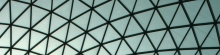## MNIST Using Keras and Convolutional Neural Networks

In a previous notebook, MNIST Using Keras, we built a fully-connected (dense) shallow neural network (one hidden layer with 512 nodes) to classify images of ...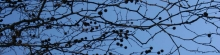## MNIST Using Keras

In this notebook, we will build a simple two-layer feed-forward neural network model using Keras, running on top of TensorFlow. We then train the sequential ...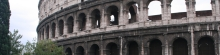## Concrete Compressive Strength Regression Model Using Keras

In this notebook, we will build a simple three-layer feed-forward neural network model using Keras, running on top of TensorFlow. The sequential model will b...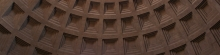## Evaluating Binary Classifier Performance